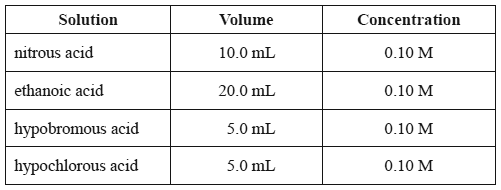Acid/base equilibria (2014 VCE) 1) The pH of the following acid solutions was measured using a pH meter.The acid solution that will have the lowest pH is A. nitrous acid. B. ethanoic acid. C. hypobromous acid. D. hypochlorous acid. Solution2) An aerosol can with a volume of 300.0 mL contains 2.80 g of propane gas as a propellant. The warning label says the aerosol may explode at temperatures above 60.0 °C. What is the pressure in the can at a temperature of 60.0 °C? A. 5.87 × 10–1 kPa B. 1.06 × 102 kPa C. 5.87 × 102 kPa D. 2.58 × 104 kPa Solution3) If Solution X has a pH of 3 and Solution Y has a pH of 6, we can conclude that A. [H+] in Solution X is 1000 times that of [H+] in Solution Y. B. [H+] in Solution X is half that of [H+] in Solution Y. C. [OH-] in Solution Y is twice that of [OH-] in Solution X. D. Solution Y must contain a stronger acid than Solution X. Solution4) What volume of 0.25 M hydrochloric acid is required to react completely with 40 mL of 0.50 M calcium hydroxide? A. 40 mL B. 80 mL C. 120 mL D. 160 mL Solution5) Two Ka values, 10–7 and 10–1, are reported for QH2. Write an equation and an expression for the acidity constant of the first ionisation reaction of QH2.   Solution 6) A 2% solution of glycolic acid (2-hydroxyethanoic acid), CH2(OH)COOH, is used in some skincare products. a) Draw the structural formula of glycolic acid. Solutionb) The equation for the ionisation of glycolic acid is CH2(OH)COOH(aq) + H2O(l) ⇌ CH2(OH)COO–(aq) + H3O+(aq) Ka = 1.48 × 10–4 Sodium glycolate, CH2(OH)COONa, is a soluble salt of glycolic acid. How does the pH of a solution of glycolic acid change when some solid sodium glycolate is dissolved in the solution? Justify your answer. Solutionc) The solubility of glycolic acid is 1.0 × 106 mg per litre at 25 °C. Calculate the concentration, in mol L–1, of a saturated solution of glycolic acid. The molar mass of glycolic acid is 76 g mol–1. Solutiond) 100 mL of the saturated solution of glycolic acid is spilt onto the floor. What is the minimum mass of sodium carbonate that should be used to neutralise the spill? The equation for this reaction is shown below. Na2CO3(s) + 2CH2(OH)COOH(aq) => 2CH2(OH)COONa(aq) + H2O(l) + CO2(g) (M(Na2CO3) = 106 g mol–1) Solutione) The Material Safety Data Sheet (MSDS) for a concentrated solution of glycolic acid states that it is corrosive to the eyes, skin and respiratory system, and that it is harm ful if a concentrated solution of it is ingested or inhaled. Outline one safety precaution that should be taken when handling this compound. Solution